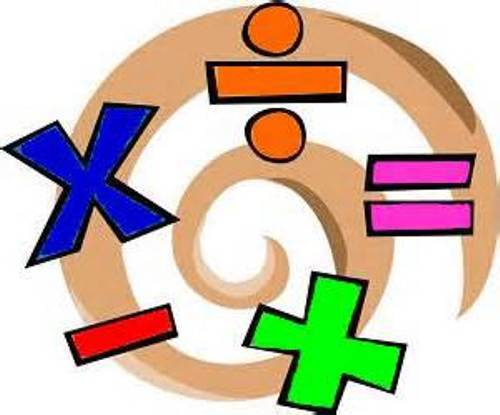Math Vocabulary
3 years ago
mrstbridge
Save
Edit
Host a game
Live GameLive
Homework
Solo Practice
Practice20 QuestionsShow answers
• Question 1
30 secondsQ. The combining of two groups into one group is called
diameter
subtraction
sdifference
• Question 2
30 secondsQ. The numbers that are added together in an addition problem are called
factors
pikachu
• Question 3
30 secondsdifference
quotient
product
sum
• Question 4
30 secondsQ. Numbers that tell position or order are called
difference
ordinal numbers
denominators
• Question 5
30 secondsQ. Taking a number away from a larger amount is called
subtraction
multiplication
• Question 6
30 secondsQ. The answer to a subtraction problem is called the
difference
sum
quotient
product
• Question 7
30 seconds
Q. Numbers that end with 2, 4, 6, 8, or 0 are
odd numbers
even numbers
ordinal numbers
pikachu
• Question 8
30 seconds
Q. Numbers that end with 1, 3, 5, 7 or 9 are called
odd numbers
even numbers
ordinal numbers
pikachu
• Question 9
30 secondsQ. The distance between the center of a circle and the outside of the circle is called the
distance
center
diameter
• Question 10
30 secondsQ. The distance across the circle through the center of it is called the
distance
center
diameter
• Question 11
30 secondsQ. The answer to a multiplication problem is called the
product
sum
quotient
difference
• Question 12
30 secondsQ. The numbers that are multiplied in a multiplication problem are called
missing numbers
products
factors
• Question 13
30 seconds
Q. Division is the opposite of what?
subtraction
multiplication
decimals
• Question 14
30 secondsQ. The answer to a division problem is called a __________.
difference
factor
product
quotient
• Question 15
30 seconds
Q. Another symbol for the division sign is what?
-
X
=
/
• Question 16
30 secondsQ. In long division, what do we call the extra number that is left over?
numerator
factor
remainder
divisor
• Question 17
30 seconds
Q. The number used to divide by in a division problem is called the:
quotient
dividend
product
divisor
• Question 18
30 secondsQ. The number being divided in a division problem is called the:
dividend
product
divisor
quotient
• Question 19
30 secondsQ. Two shapes that are the same shape, but different sizes are called
congruent
similar
perpendicular
equal
• Question 20
30 secondsQ. Two figures that are the same shape AND the same size are called